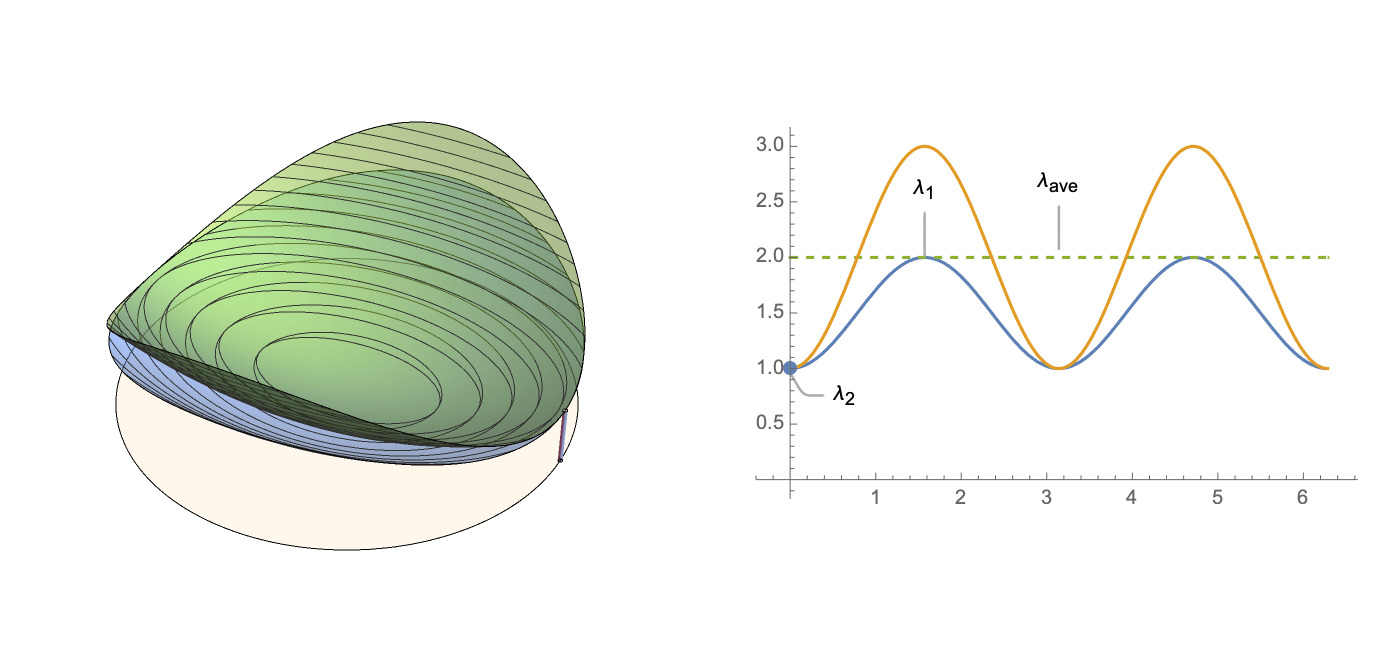# #StackBounty: #normal-distribution #circular-statistics Expected ratio of x'Ax and x'AAx on a unit sphere?

### Bounty: 50

Suppose $$A$$ is symmetric positive definite matrix. Is there a nice expression for the first moment of the following quantity?

$$frac{x^TAx}{x^TA^2 x}$$

Where $$x$$ is distributed as $$text{Normal}(0,I_n)$$. This is the ratio of two quadratic forms evaluated on the surface of the sphere.When $$A$$ has eigenvalues $$langle 1, frac{1}{2}rangle$$, this expectation is equal to $$frac{4}{3}$$

Get this bounty!!!

This site uses Akismet to reduce spam. Learn how your comment data is processed.